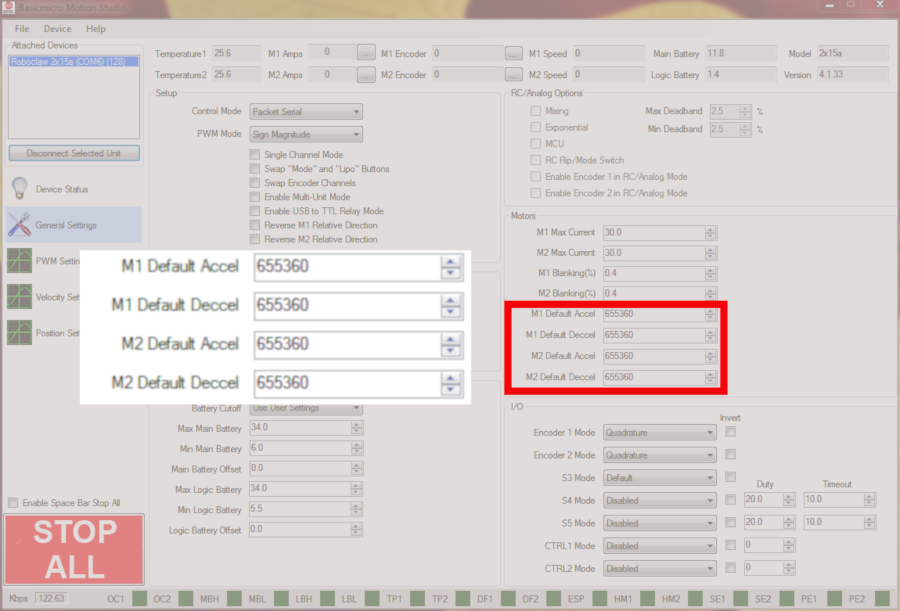# Understanding Acceleration and Deceleration Values

This Application Note takes a look at how to calculate acceleration and deceleration values for use in Motion Studio and with the available software libraries. The first thing to understand is that the value that is used for acceleration or deceleration is unit-less and does not correspond to encoder counts like many of the other settings. The values that is used for either acceleration or deceleration can be thought of it three different terms: the raw value, as a percentage and as a time value.

The raw value is what is entered in to the fields in Motion Studio and in the commands in the software libraries. The default value in the “Default Acceleration” and “Default Deceleration” fields in Motion Studio is 655360. This value can be tweaked by trial and error until a satisfactory values is reached. However, looking at this value in terms of time or percentage is a bit more useful.

To look at the value in terms of percentage it should be understood that the value of 32768 is equal to 100%. To convert a raw value to a percentage divide the value by 32768 and multiply the result by 100. To convert from a percentage to a value divide the percentage by 100 and the multiply the result by 32768.

Calculate percentage:

percentage = ( raw value / 32768 ) x 100

Calculate raw value from percentage:

raw value = ( percentage / 100 ) x 32768

Another way of looking at a raw value is in terms of time. With a value of 32768 or a percentage of 100% the motor controller will reach the target value in one second. See the formulas below to see how to convert from one value to another.

Calculate time from raw value:

time (in seconds) = ( raw value / 32768 ) x 1

Calculate raw value for a given time:

raw value = 32768 / time in secondsFigure 1: Location of default acceleration and deceleration values in Motion Studio.